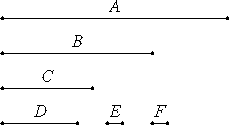# Proposition 4

To find the greatest common measure of three given commensurable magnitudes.

Let A, B, and C be the three given commensurable magnitudes.

It is required to find the greatest common measure of A, B, and C.

X.3

Take the greatest common measure D of the two magnitudes A and B.

Either D measures C, or it does not measure it.

First, let it measure it.

Since then D measures C, while it also measures A and B, therefore D is a common measure of A, B, and C. And it is manifest that it is also the greatest, for a greater magnitude than the magnitude D does not measure A and B.Next, let D not measure C.

I say first that C and D are commensurable.

X.3.Cor.

Since A, B, and C are commensurable, some magnitude measures them, and this of course measures A and B also, so that it also measures the greatest common measure of A and B, namely D.

But it also measures C, so that the said magnitude measures C and D, therefore C and D are commensurable.

X.3

Now take their greatest common measure E.

Since E measures D, while D measures A and B, therefore E also measures A and B. But it measures C also, therefore E measures A, B, and C. Therefore E is a common measure of A, B, and C.

I say next that it is also the greatest.

For, if possible, let there be some magnitude F greater than E, and let it measure A, B, and C.

X.3,Cor.

Now, since F measures A, B, and C, it also measures A and B, and therefore measures the greatest common measure of A and B.

But the greatest common measure of A and B is D, therefore F measures D.

X.3,Cor.

But it measures C also, therefore F measures C and D. Therefore F also measures the greatest common measure of C and D. But that is E, therefore F measures E, the greater the less, which is impossible.

Therefore no magnitude greater than the magnitude E measures A, B, and C. Therefore E is the greatest common measure of A, B, and C if D does not measure C, but if it measures it, then D is itself the greatest common measure.

Therefore the greatest common measure of the three given commensurable magnitudes has been found.

Q.E.D.

# Corollary.

From this it is clear that, if a magnitude measures three magnitudes, then it also measures their greatest common measure. The greatest common measure can be found similarly for more magnitudes, and the corollary extended.

## Guide

This is the same proposition as VII.3. This proposition and the last explain how to find the common measure of commensurable magnitudes. Although not explicitly invoked, they bear on the succeeding propositions which use common measures of commensurable magnitudes.